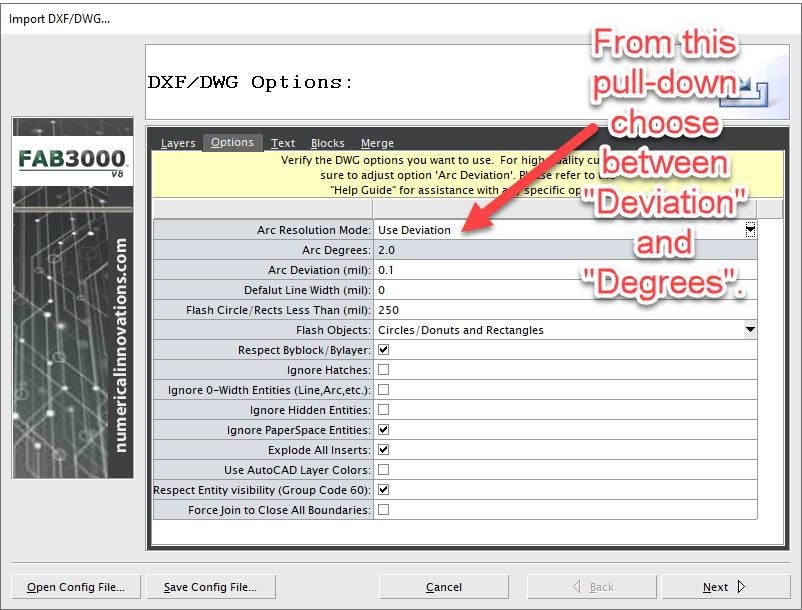Start a new topic

## Imported DXF circles have different # of segments.

During DXF/DWG Import, FAB 3000 allows you to choose what accuracy you wish to use when outputting arcs: Resolution (Degrees) or Deviation (Tolerance).Arc Resolution (Degrees):

Lets you choose a degree (such as 3), and then each circle regardless of the diameter will be converted with 120 segments. If you have a small circle, 120 segments will probably be too many vertices causing overlapping vertices, and wasted data. If you have a circle too large, 120 segments may not be enough and the circle will look segmented (i.e. more like a "Stop Sign" than a true circle).  You will typically always get the same number of segments no matter what radius is used.

Arc Deviation (Tolerance).
Lets you define a tolerance for your arcs. Thus if you set a value of 0.1 mil for your deviation X number of segments will be added to insure your circle remains with in tolerance. With Arc deviation the number of vertex (segments) for a circle will vary for two reasons:

(1) Diameter of the Circle
(2) Value for the Arc Deviation

Note: The arc deviation controls the "tangent distance" from the DXF arc/circle to the polygon segment. The smaller the "Arc Deviation" the more vertex (segments) that will be used in the resulting polygon. What also controls the number of vertex (segments) is the diameter of the circle. If a circle has a large diameter it will contain more segments than a circle with a smaller diameter. The advantage of using "Arc Deviation" is that FAB 3000 will intelligently optimize each circle to use the perfect amount of vertices - which results in reduced data size (for small circles) & improves overall quality (for large circles).